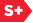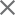### Proceedings Paper

Thresholding in a calculation algorithm for fast approximation of wavelet transform
Author(s): Charles C. Chang; Jyh-Charn S. Liu; Andrew K. Chan
Format Member Price Non-Member Price
PDF \$17.00 \$21.00

Paper Abstract

Wavelet transform has been widely used in many signal and image processing applications such as edge detection and data compression. For applications that tolerate a slight compromise in accuracy for faster speed, a fast approximation of wavelet transform is favorable. In this paper we propose a simple yet effective algorithm for fast wavelet transform. The use of fixed point numbers simplifies the hardware design and computational complexity than the use of a floating point arithmetic unit. Calculations are further reduced using our thresholding-in-calculation (TIC) technique to omit calculations of small terms that are negligible to the accumulated sum. The TIC technique basically determines whether a multiplication followed by an addition shall be executed using a look-up table with the quantized magnitude of multiplication operands as input parameters. Knowing the levels of quantization, any combination of the quantized multiplication operands can approximate the product and be compared to a predetermined threshold value. If the approximated product is greater than or equal to the threshold value, the corresponding entry in the look-up table is marked for multiplication; otherwise, no multiplication will be executed. Our simulation results show that our approximation algorithm is effective for wavelet transform of audio signals. In addition, when our algorithm is applied to a simple wavelet based edge detection algorithm, the detection result is almost the same as the one using precise calculation of the wavelet transform.

Paper Details

Date Published: 30 October 1997
PDF: 8 pages
Proc. SPIE 3169, Wavelet Applications in Signal and Image Processing V, (30 October 1997); doi: 10.1117/12.292807
Show Author Affiliations
Charles C. Chang, Texas A&M Univ. (United States)
Jyh-Charn S. Liu, Texas A&M Univ. (United States)
Andrew K. Chan, Texas A&M Univ. (United States)

Published in SPIE Proceedings Vol. 3169:
Wavelet Applications in Signal and Image Processing V
Akram Aldroubi; Andrew F. Laine; Michael A. Unser, Editor(s)